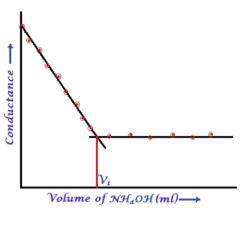Courses

# Test: Electrochemistry - 1

## 20 Questions MCQ Test Physical Chemistry | Test: Electrochemistry - 1

Description
This mock test of Test: Electrochemistry - 1 for Chemistry helps you for every Chemistry entrance exam. This contains 20 Multiple Choice Questions for Chemistry Test: Electrochemistry - 1 (mcq) to study with solutions a complete question bank. The solved questions answers in this Test: Electrochemistry - 1 quiz give you a good mix of easy questions and tough questions. Chemistry students definitely take this Test: Electrochemistry - 1 exercise for a better result in the exam. You can find other Test: Electrochemistry - 1 extra questions, long questions & short questions for Chemistry on EduRev as well by searching above.
QUESTION: 1

### The standard reduction potential at 298 K for the following half cells are given: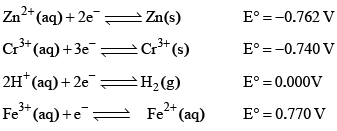Which is the strongest reducing agent:

Solution:

Zn has minimum reduction potential it means Zn is strong reducing agent. Hence A is correct.

QUESTION: 2

Solution:
QUESTION: 3

### The electric charge for electrode deposition of one gram equivalent of a substance is:

Solution:
QUESTION: 4

The standard reduction potentials E°, for the half reactions are as: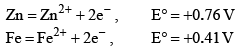The emf for the cell reaction,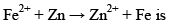Solution: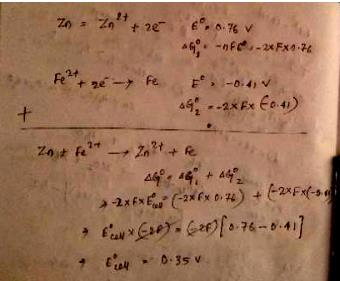QUESTION: 5

The correct order of equivalent conductance at infinite dilution of LiCl, NaCl and KCl is:

Solution:

The correct order of equivalent conductance at infinite dilution is KCl > NaCl > LiCl.

Anion is same (chloride ion) for all the species. Larger is the size of the cation, greater is the equivalent conductance at infinite dilution and vice versa.

QUESTION: 6

Saturated solution of KNO3 is used to make ‘salt-bridge’ because:

Solution:
QUESTION: 7

Standard electrode potential data are useful for understanding the suitability of an oxidant in a redox titration. Some half-cell reactions and their standard potentials are given below: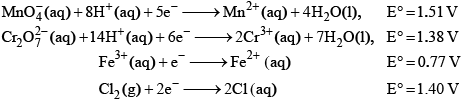Identify the incorrect statement regarding the quantitative estimation of aqueous Fe(NO3)2

Solution:
QUESTION: 8

In the electrolytic cell, flow of electrons is from:

Solution:
QUESTION: 9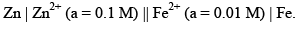The emf of the above cell is 0.2905 V. Equilibrium constant for the cell reaction is:

Solution: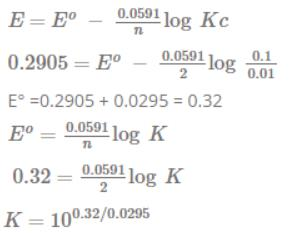QUESTION: 10

If standard potential of the reaction 2H2O(l) = O2(g, 1bar) + 4H+(aq) + 4e is E° = –1.23 V. At 25°C and pH = 7, what is the oxidation potential of pure water according to this reaction:

Solution:
QUESTION: 11

Based on the data given blow strongest oxidizing agent will be: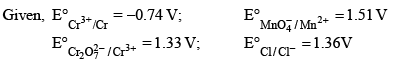Solution:

As MnO4- has highest reduction potential among them so it is strongest oxidizing agent.

QUESTION: 12

An aqueous solution of X is added slowly to an aqueous solution of Y as shown in List I. The variation in conductivity of these reactions is given in List II. Match List I with List II and select the correct answer using the code given below the lists.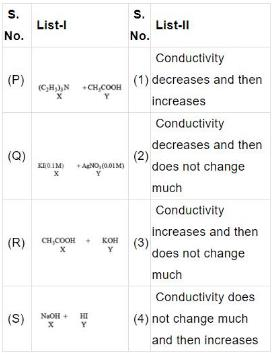Codes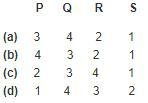Solution: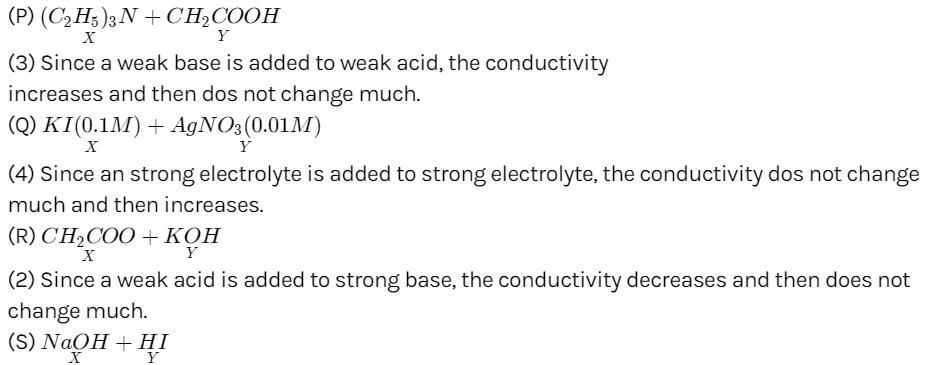(1) Since a strong base is added to an acid, the conductivity decreases and then increases.

QUESTION: 13

The electrochemical cell shown below is a concentration cell.
M|M2+ (saturated solut ion of a sparingly so luble salt, MX2 || M2+ (0.001 mol dm–3)| M.
The emf of the cell depends on the difference in concentration of M2+ ions at the two electrodes. The emf of the cell at 298  is 0.059 V.

Q.

The solubility product (Ksp ; mol3 dm–9) of MX2 at 298 based on the informat ion available the given concentration cell is (take 2.303 × R × 298/F = 0.059 V)

Solution:
QUESTION: 14

The electrochemical cell shown below is a concentration cell.
M|M2+ (saturated solut ion of a sparingly so luble salt, MX2 || M2+ (0.001 mol dm–3)| M.
The emf of the cell depends on the difference in concentration of M2+ ions at the two electrodes. The emf of the cell at 298  is 0.059 V.

Q.

The value of ΔG (kJ mol-1) for the given cell is (take 1 F = 96500 C mol-1)

Solution:

At cathode:M+2(aq)+2e→M(s)
At anode:M(s)+2X(aq)→MX2​(aq)+2e
n - factor of the cell reaction is 2.

ΔG=−nFEcell​

= −2×96500×0.059

= −11.4 kJmole−1

QUESTION: 15

For the following cell,

Zn(s) | ZnSO4(aq) || CuSO4(aq) | Cu(s)

When the concentration of Zn2+ is 10 times the concentration of Cu2+, the expression for ΔG (in J mol-1) is [F is Faraday constant; R is gas constant; T is temperature; E° (cell) = 1.1 V]

Solution: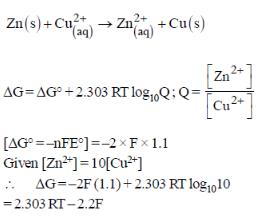QUESTION: 16

Galvanization is applying a coating of:

Solution:
QUESTION: 17

An electrochemical cell consists of two half-cell reactions.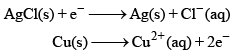The mass of copper (in grams) dissolved on passing 0.5 A current for 1h is [Given, atomic mass of Cu is 63.6, F = 96500 C mol–1]

Solution:

m/E = Q/F
m/(63.6/2) = (0.5 x 60 x 60)/96500
m = (1800 x 63.6)/(96500 x 2)
m = 0.59

QUESTION: 18

In the following Latimer diagram, the species that undergoes disproportionation reaction is: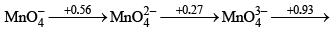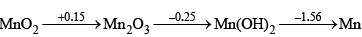Solution:

This can be explained with the help of Latimer diagram which gives us information in a compact form about the Eo(reduction potential) for the conversion of different oxidation States of an element. "A species has a tendency to disproportionate to its nearest neighbours if the potential on right of the species in the diagram is higher than the potential on the left". According to this diagram only MnO4³- has high potential on right side than left side so it only has tendency to disproportionate into MnO4²- & MnO2

Hence the correct answer is B.

QUESTION: 19

On titrating conduct metrically a NaOH solution with a mixture of HCl and CH3CO2H solutions, plot of the volume of mixed acid added (b) in y-axis against the conductance (a) in x-axis is expected to look like:

Solution:

The titration occur between mixture HCl and CH3COOH solution (strong acid and weak acid) with NaOH solution (strong base).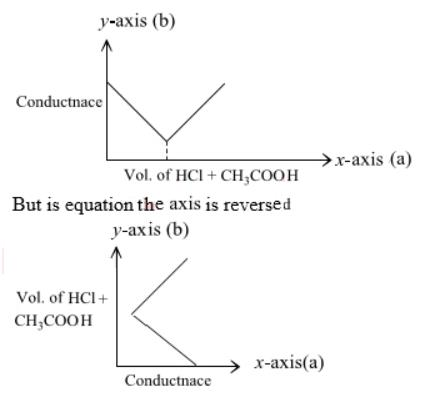QUESTION: 20

In a typical Conductometric titration of a strong acid with a weak base, the curve resembles:

Solution:

Here consider a strong acid as HCL and weak base as Ammonium hydroxide that is NH4OH. Suppose the acid has high concentration of H positive ions due to which it show high conductance, when a weak base is added to it, the cation of weak base combines with Cl Negative ion to form ammonium chloride precipitate thus decreasing the conductivity by utilizing H positive ion to form water molecule due to this decrease in conductivity the graph shows on negative fall in conductance after all the edge positive and Cl negative ions are combine end point is reached where no free ions are possible for conduction after this when the basis added to form NH4 positive and was OH negative since it is a weak base it has low conductivity and the graph increases very slowly towards conduction minute changes in increasing conduction can be seen when a weak bases are added

plot a) show high conductivity which is not possible in weak bases
plot c) and d) involve 3 mixture components which is not the case
The perfect diagram would look like this and B is the closest.